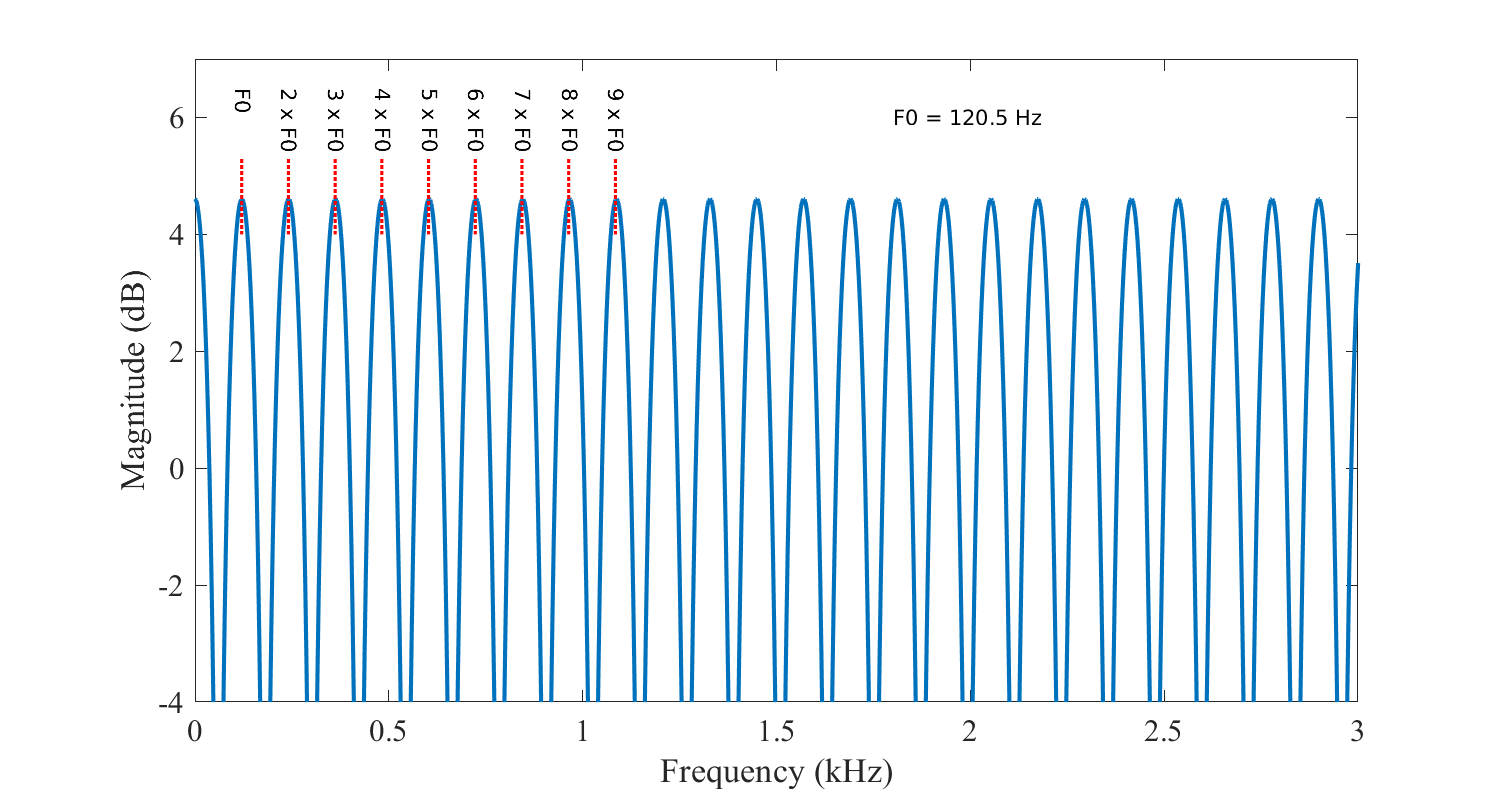# 3.10. Fundamental frequency (F0)#

The fundamental frequency of a speech signal, often denoted by F0 or $$F_{0}$$, refers to the approximate frequency of the (quasi-)periodic structure of voiced speech signals. The oscillation originates from the vocal folds, which oscillate in the airflow when appropriately tensed. The fundamental frequency is defined as the average number of oscillations per second and expressed in Hertz. Since the oscillation originates from an organic structure, it is not exactly periodic but contains significant fluctuations. In particular, amount of variation in period length and amplitude are known respectively as jitter and shimmer. Moreover, the F0 is typically not stationary, but changes constantly within a sentence. In fact, the F0 can be used for expressive purposes to signify, for example, emphasis and questions.

Typically fundamental frequencies lie roughly in the range 80 to 450 Hz, where males have lower voices than females and children. The F0 of an individual speaker depends primarily on the length of the vocal folds, which is in turn correlated with overall body size. Cultural and stylistic aspects of speech naturally have also a large impact.

The fundamental frequency is closely related to pitch, which is defined as our perception of fundamental frequency. That is, the F0 describes the actual physical phenomenon, whereas pitch describes how our ears and brains interpret the signal, in terms of periodicity. For example, a voice signal could have an F0 of 100 Hz. If we then apply a high-pass filter to remove all signal components below 450 Hz, then that would remove the actual fundamental frequency. The lowest remaining periodic component would be 500 Hz, which correspond to the fifth harmonic of the original F0. However, a human listener would then typically still perceive a pitch of 100 Hz, even if it does not exist anymore. The brain somehow reconstructs the fundamental from the upper harmonics. This well-known phenomenon is however still not completely understood.

A speech signal with a fundamental frequency of approximately F0=93Hz.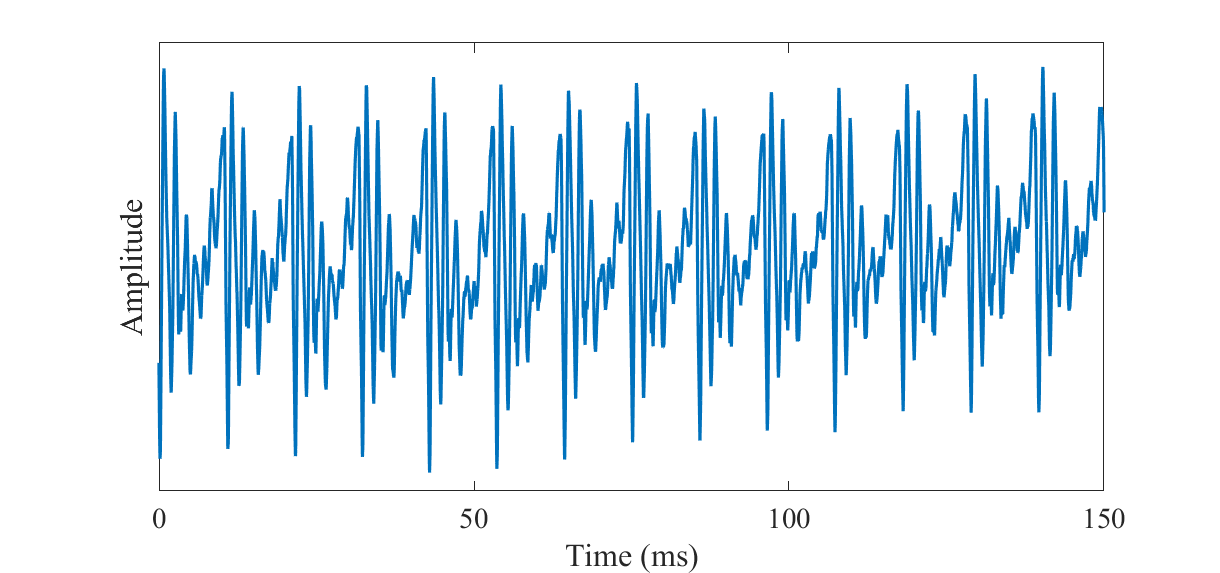The spectrum of a speech signal with a fundamental frequency of approximately F0=93Hz (original) and a high-pass filtered version of it such that the fundamental frequency has been removed (high-pass filtered).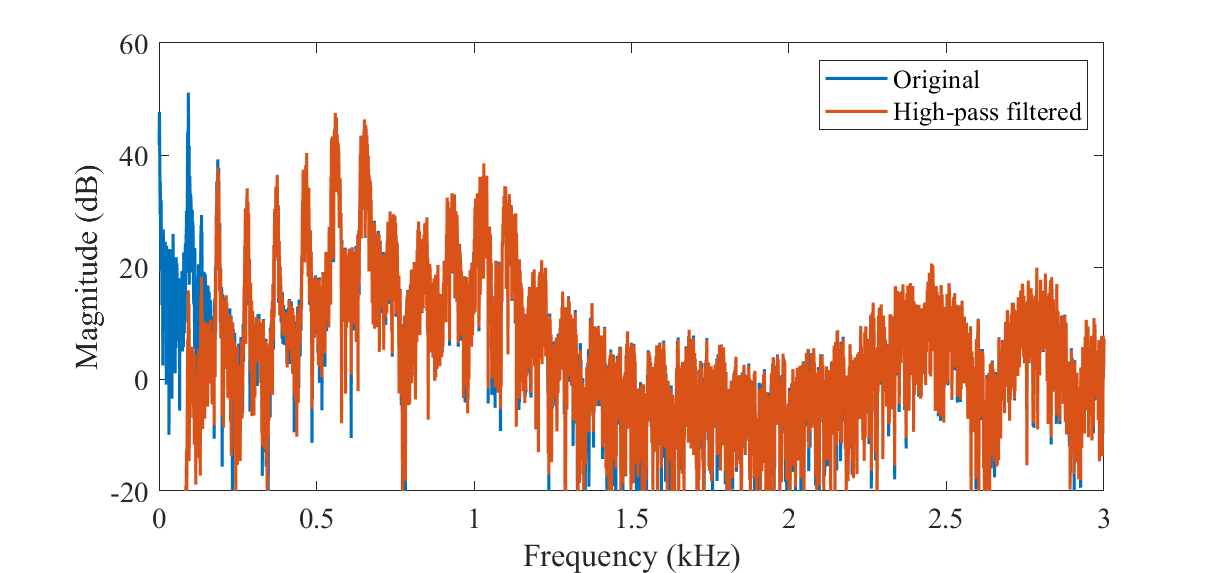A speech signal with a fundamental frequency of approximately F0=93Hz

Hide code cell source
import IPython.display as ipd
ipd.Audio('attachments/175515683.wav')


A high-pass filtered version of it such that the fundamental frequency has been removed

Hide code cell source
import IPython.display as ipd
ipd.Audio('attachments/175515684.wav')


If $$F_{0}$$ is the fundamental frequency, then the length of a single period in seconds is

$T=\frac{1}{F_0}.$

The speech waveform thus repeats itself after every $$T$$ seconds.

A simple way of modelling the fundamental frequency is to repeat the signal after a delay of $$T$$ seconds. If a signal is sampled with a sampling rate of $$F_{s}$$, then the signal repeats after a delay of $$L$$ samples where

$L = F_s T = \frac{F_s}{F_0}.$

A signal $$x_{n}$$ then approximately repeats itself such that

$x_n \approx x\_{n-L} \approx x\_{n-2L} \approx x\_{n-3L}.$

In the Z-domain this can be modelled by an IIR-filter as

$B(z) = 1 - \gamma_L z^{-L},$

where the scalar $$0\leq\gamma_L\leq 1$$ scales with the accuracy of the period. The Z-transform of the signal $$x_{n}$$ can then be written as $$X(z)=B^{-1}(z) E(z),$$ where $$E(z)$$ is the Z-transform of a single period.

Segment of a speech signal, with the period length $$L$$, and fundamental frequency $$F_0=1/L$$.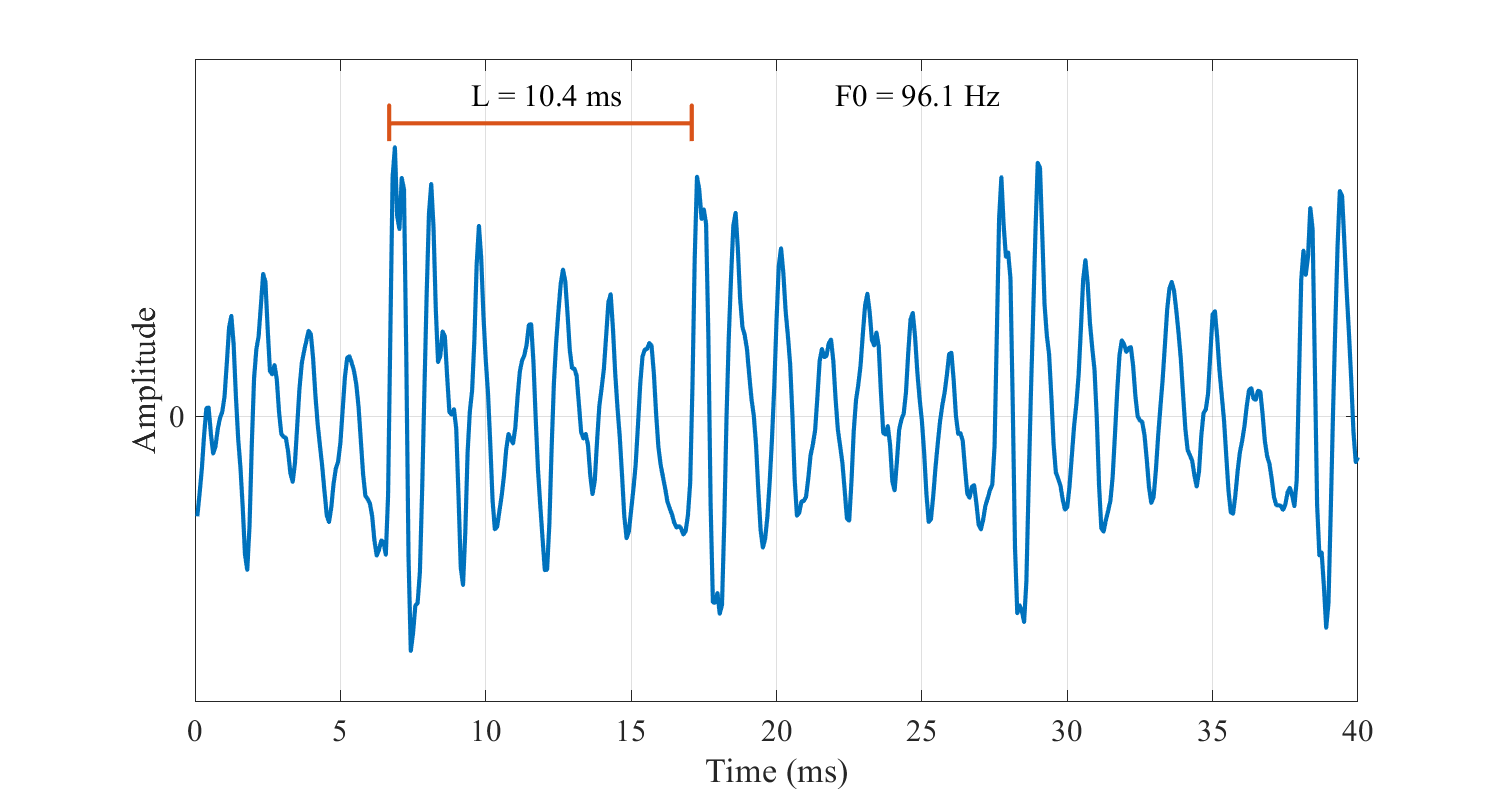Spectrum of speech signal with the fundamental frequency $$F_{0}$$ and harmonics $$kF_{0}$$, as well as the formants F1, F2, F3… Notice how the harmonics form a regular comb-structure.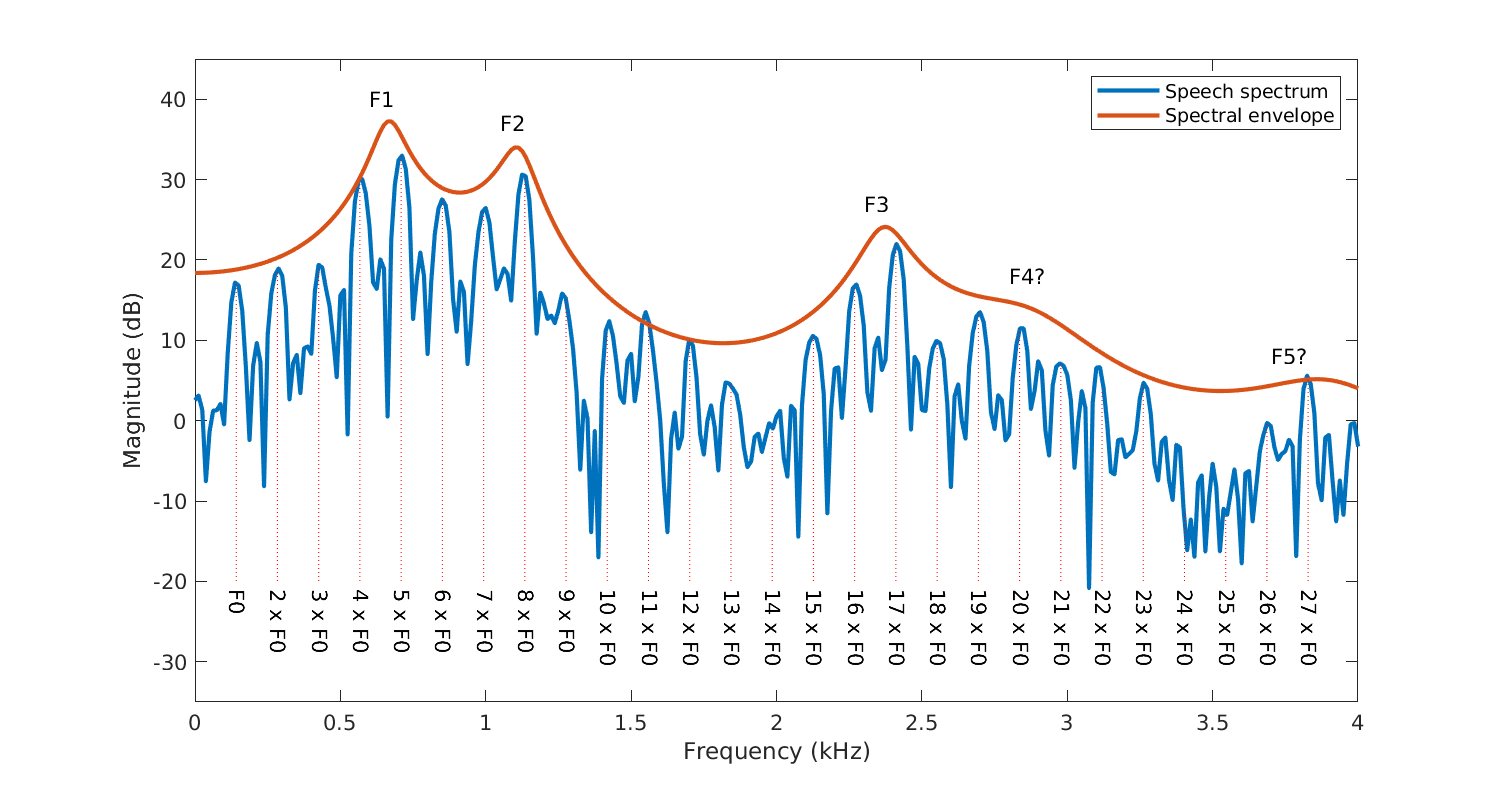The magnitude spectrum of $$B^{-1}(z)$$, has then a periodic comb-structure. That is, the magnitude spectrum has peaks at $$k\,F_0$$ , for integer $$k$$. For a discussion about the fundamental frequency in the cepstral domain, see Cepstrum and MFCC.

Spectrum of fundamental frequency model $$B^{-1}(z)$$, showing the characteristic comb-structure with harmonic peaks appearing at integer multiples of $$F0$$.